Latest Banking jobs   »   Quantitative Aptitude Quiz For SBI PO/Clerk...

# Quantitative Aptitude Quiz For SBI PO/Clerk Mains 2023- 1st January

Q1. First number is 300% more than the second number and third number is 200% more than the second number. First number is 150% of the fourth number. which of the following is always true?
(a) The first number is divisible by 24.
(b) The second number is divisible by 9.
(c) The product of fourth and any of the three numbers is divisible by 24.
(d) The third number is divisible by 18.
(e) None of the above.

Directions (2-5): In the given questions, two quantities are given, one as ‘Quantity I’ and another as ‘Quantity II’. You have to determine relationship between two quantities and choose the appropriate option:

Q2. Quantity I – A can complete a task in 24 days and B can do same task in 18 days. And C & D together complete the same task in 12 days and ratio of efficiency of D to that of C is 7: 5. Find in how many days A, B and D together will be complete that work?
Quantity II – A is 60% less efficient than S and can complete a piece of work in 22.5 days. A and S start the work together and after 4.5 days both left the work, if B complete the remaining of work in 4.5 days then find in how many days the whole work will be completed, if all three work together?
(a) Quantity I > Quantity II
(b) Quantity I < Quantity II
(c) Quantity I ≥ Quantity II
(d) Quantity I ≤ Quantity II
(e) Quantity I = Quantity II or no relation

Q3. Quantity I – A bag contains four green balls, three red balls and five blue balls. If three balls taken out at random what is probability of getting at least one green ball & one blue ball.
Quantity II – There are five red toys and six green toys in a carton. If four toys are drawn at random then what is the probability of getting at least two green toys?
(a) Quantity I > Quantity II
(b) Quantity I < Quantity II
(c) Quantity I ≥ Quantity II
(d) Quantity I ≤ Quantity II
(e) Quantity I = Quantity II or no relation

Q4. Quantity I – Six years ago, age of A is 12½% less than the age of B, while six years later, ratio of age of A to that of B will be 9 : 10. The age of A two years later will be?
Quantity II – Ratio between age of A, B and C is 16 : 9 : 7. Three years hence average of all three age will be 35 years. The age of A two years hence will be?
(a) Quantity I > Quantity II
(b) Quantity I < Quantity II
(c) Quantity I ≥ Quantity II
(d) Quantity I ≤ Quantity II
(e) Quantity I = Quantity II or no relation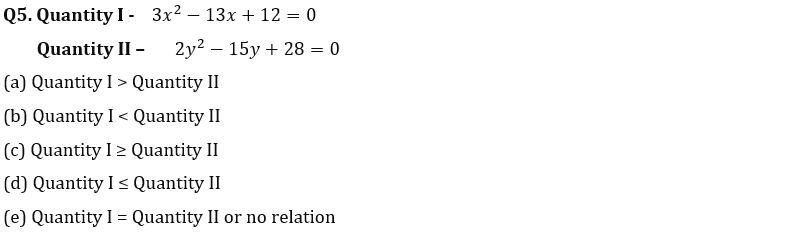Directions (6–9): Read the following information carefully and answer the following questions.
There are 3 societies A, B, C in an area. There are 300 males in society A which are 20 less than males in society C. Ratio of females in society B to that of in society C is 1 : 2. There are 620 females in all 3 societies together. Total number of residents in society C are 20% more than that of in society A.

Q6. What is difference between number of females in society A and in society C?
(a) 100
(b) 70
(c) 60
(d) 90
(e) 80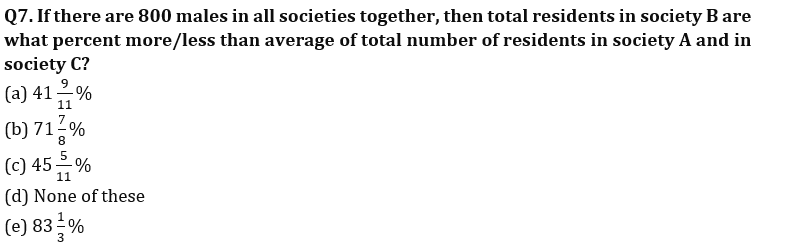Q8. If total males in all societies together are at least 30% more than total females in all societies together, then what can be the ratio of males to females in society B?
A. 93 : 70 B. 19 : 14 C. 9 : 7 D. 39 : 28 E. 37 : 28
(a) A, C, D
(b) A, B, D
(c) B, D, E
(d) C, D, E
(e) A, C, E

Q9. How many residents are in society B if the difference between males and females is minimum & maximum for society C & society A respectively?
(a) 400
(b) 380
(c) 320
(d) 360
(e) 300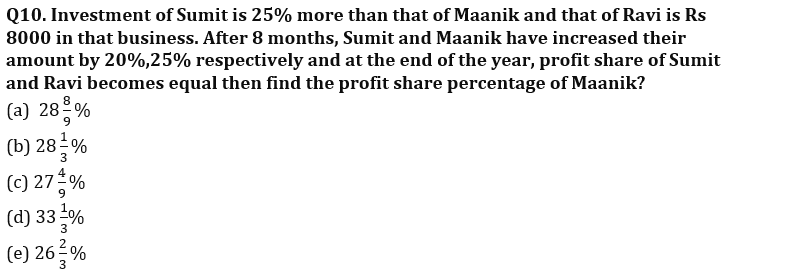Directions (11-15): Find the wrong number in the following number series:

Q11. 6, 4, 5, 11, 45, 189, 1127
(a) 189
(b) 6
(c) 45
(d) 1127
(e) 11

Q12. 13.2, 12.8, 13.4, 12.6, 14.2, 11, 17.4
(a) 12.8
(b) 12.6
(c) 13.2
(d) 11
(e) 17.4

Q13. 1130, 1128, 1108, 1072, 1004, 872, 612
(a) 1072
(b) 872
(c) 1108
(d) 1130
(e) 612

Q14. 280, 280, 286, 306, 348, 420, 540
(a) 286
(b) 348
(c) 420
(d) 280
(e) 540

Q15. 676, 576, 484, 400, 340, 256, 196
(a) 196
(b) 340
(c) 676
(d) 576
(e) 400

Solutions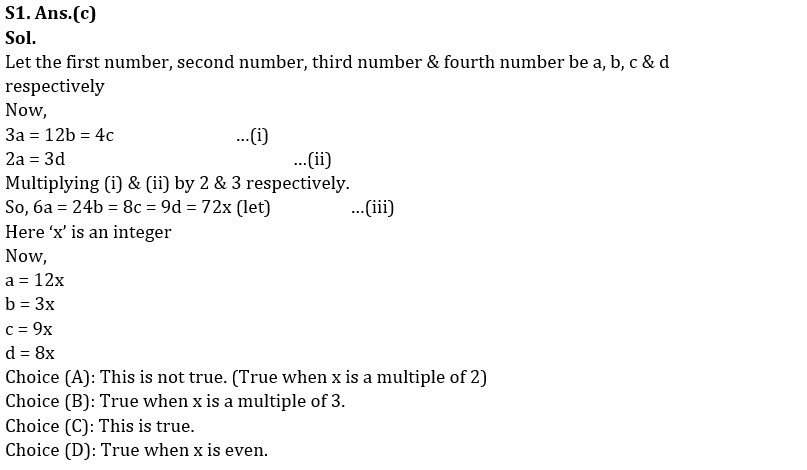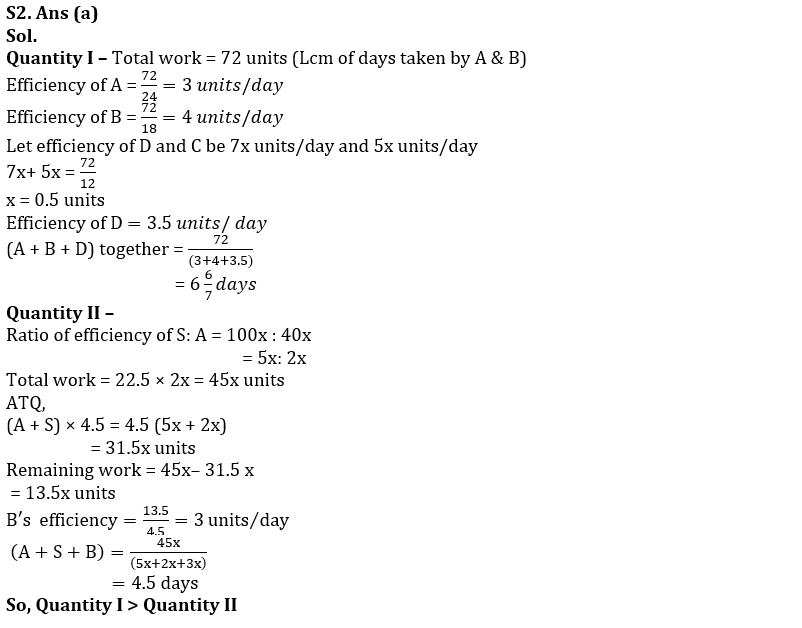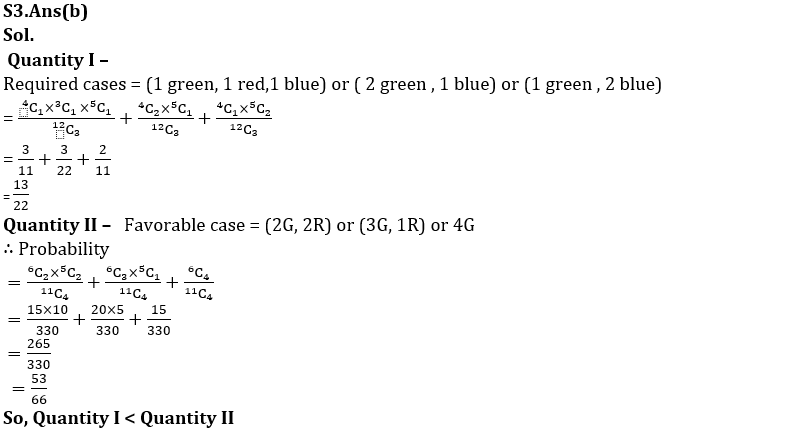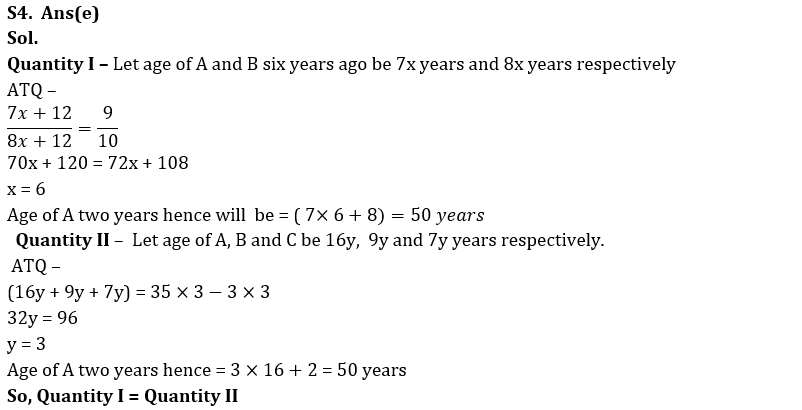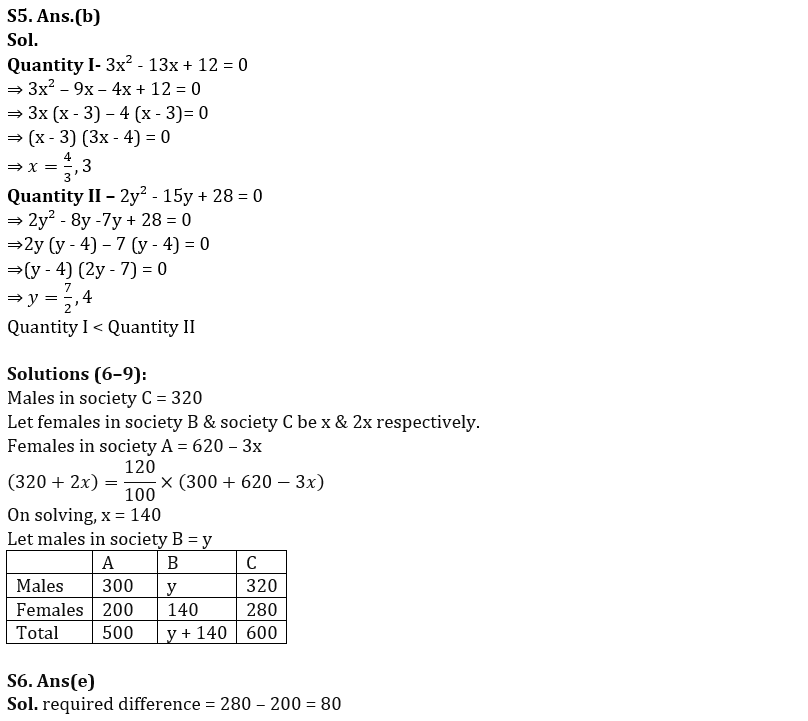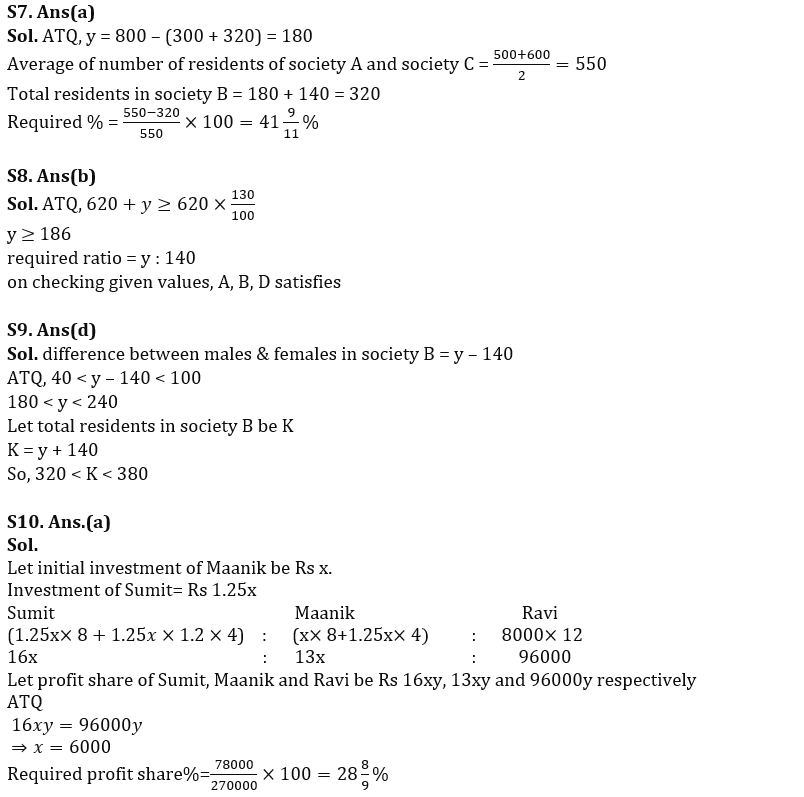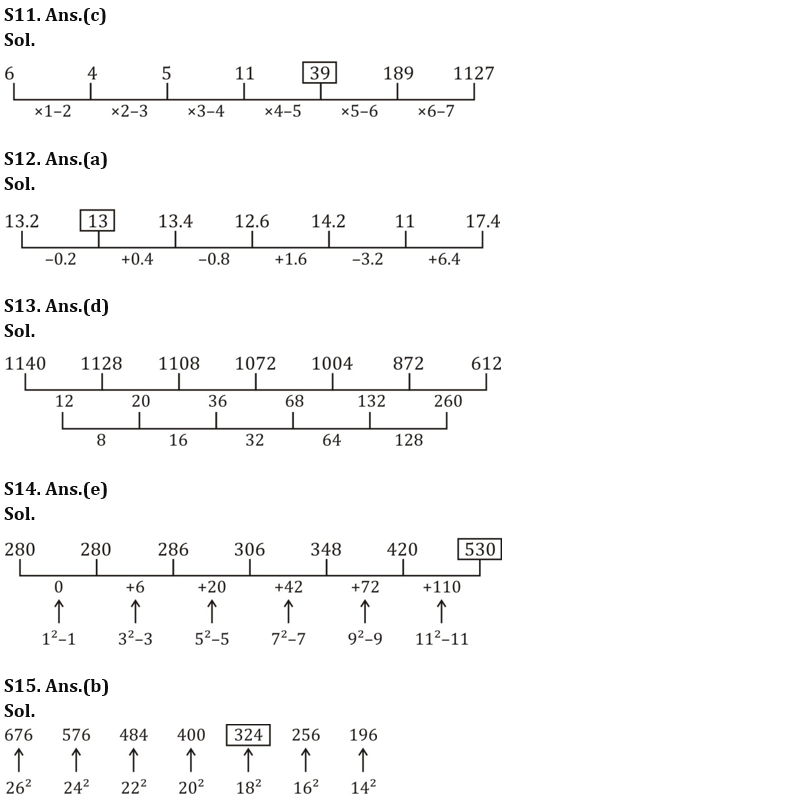#### Congratulations!